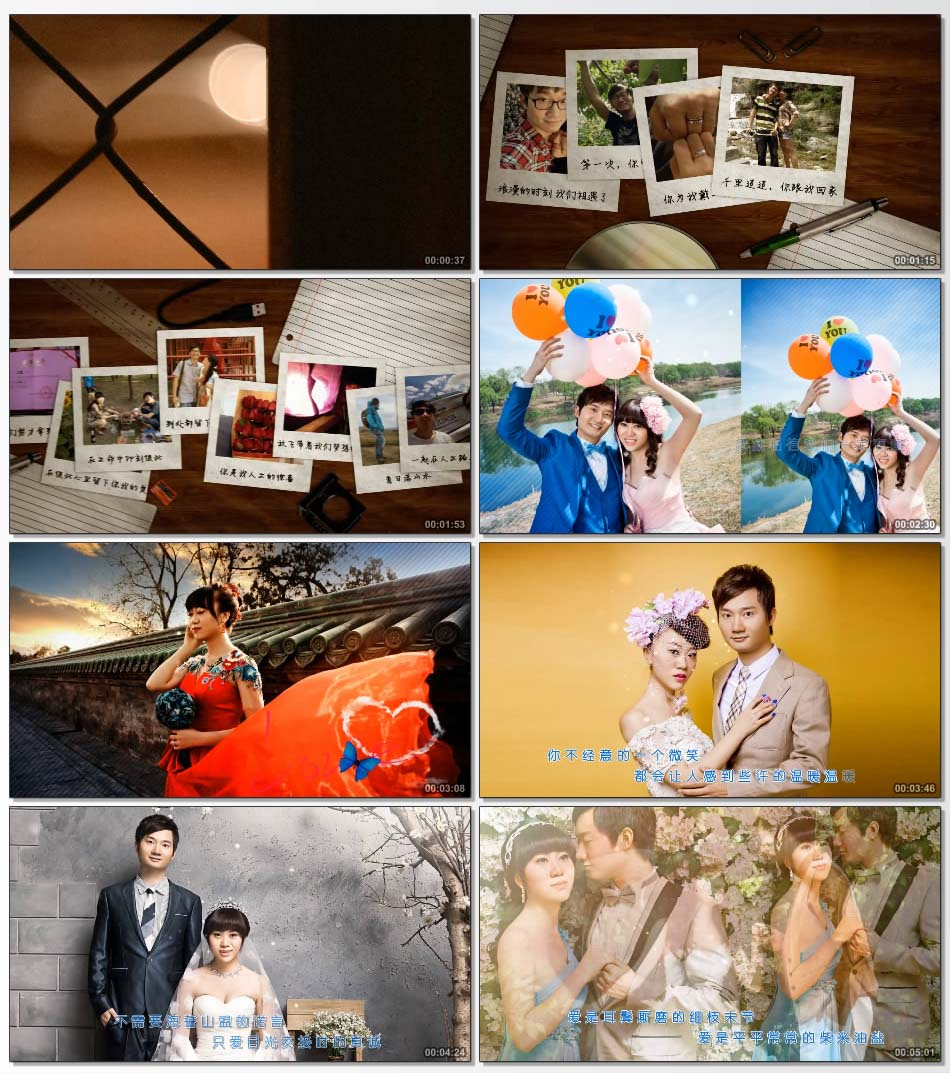# 爱在深秋t1=20--30岁和你一起浪漫
t2=30--40岁我们一起生活
t3=40--50岁我们一起平淡
t4=50--60岁我们一起变老
t5=60--70岁我们一起糊涂
t6=80岁我们再拍一次婚纱照
t7=希望下辈子还在一起
t8=浪漫的时刻 我们相遇了
t9=第一次，你带我回家
t10=你为我戴上情侣对戒
t11=千里迢迢，你跟我回家
t12=我们努力拿到的证书
t13=在彼此心里留下你我的爱
t14=在生命中印刻彼此
t15=到处都留下我们的笑声
t16=你是我人生的惊喜
t17=放飞带着我们梦想的青春
t18=看日落山水
t19=一起在人生路上旅行
t20=我们的幸福开始
t21=从相遇的那一时刻起
t22=我就相信我们一定有缘
t23=从此我的脑海脑海里，便多了一份牵挂
t24=每当看到你乖巧忙碌的身影
我的目光悄悄为你停留
t25=每当听到你清脆悦耳的声音
就让我想起童话中的百灵鸟
t26=你不经意的一个微笑
都会让人感到些许的温暖温暖
t27=不需要海誓山盟的诺言
只爱目光交接时的真诚
t28=不需要形影不离的厮守
只爱肩并肩的温暖
t29=此生，遇见你，已是很美
此生，爱上你，无怨无悔
t30=爱是耳鬓厮磨的细枝末节
爱是平平常常的柴米油盐
t31=幸福牵手
t32=秦朝&苏姬
t33=2015.12.18
t34=感谢大家见证这幸福的时刻